Next: Estimation with more than Up: Estimating camera response function Previous: Computational efficiency

### Robust statistics and yet more computationally efficient solutions: From  Lebesgue summation'' to comparagram

Another way to interpret the error presented in (9) is to rewrite the summation as a sum over the range rather than over the domain of the images4: = _m=0^N _n=0^N J(m,n) ( F(f_1(x,y)) - F(f_2(x,y)) + K ) ^2 summing over the values of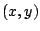for which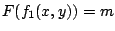and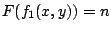.

The quantity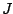provides a weighting for the number of pixels in image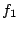that are equal to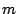and at the same coordinatesequal to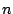in image.

It will be easier to understand the form of (15) by grouping (counting) all of the pixel values for which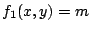and, in the corresponding location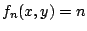. The result, denoted by the letter, is called the comparagram of the two images, and has dimensionsby. It should be noted thatcaptures all of the information about the tonal relationship between the two pictures while discarding all of the spatial information in the images. This tonal relationship is all that is needed to solve (9) while discarding a great deal of irrelevant information. To solve (15), the matrix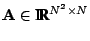and the vector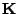are constructed to have one row for each element of, as follows: A(mN+n,m) &=&J(m,n)
A(mN+n,n) &=&-J(m,n)
K(mN+n) &=&J(m,n) with the additional constraints appended to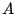as before, resulting in the same method of solution as before (least-squares).

Looking at comparagrams of typical sets of differently exposed images, the comparagrams tend to be very sparse. Thus working with comparagrams provides us with a very tidy way of greatly increasing the computational efficiency, as well as performing robust statistics (pruning low entries).

Thus the computation can be made still more efficient by removing rows ofand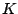that are zero, which is done by only including nonempty bins ofthat are off the main diagonal (zero entries result from either empty bins ofor from diagonal entries of). Jointly, the comparagram across two images seldom contains more than ten percent nonzero entries, so the resulting computational savings is very significant.

Robust statistics provide improved immunity to noise but this immunity is usually obtained at some computational expense. However, the formulation of (15) with solution (17) may be made both computationally more efficient as well as more robust by thresholding. This is done by setting any entries inthat are less than a certain number equal to zero. A threshold of 10, for example, means that any bins containing less than 10 counts are set to zero prior to solving (17). Interestingly enough, this method of throwing away outliers results in further increases in computational efficiency.Next: Estimation with more than Up: Estimating camera response function Previous: Computational efficiency
Steve Mann 2002-05-25